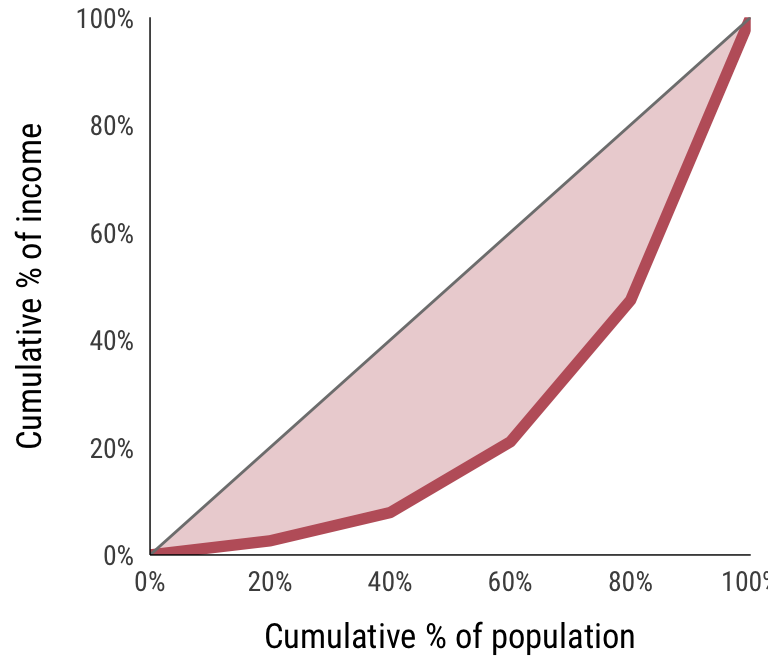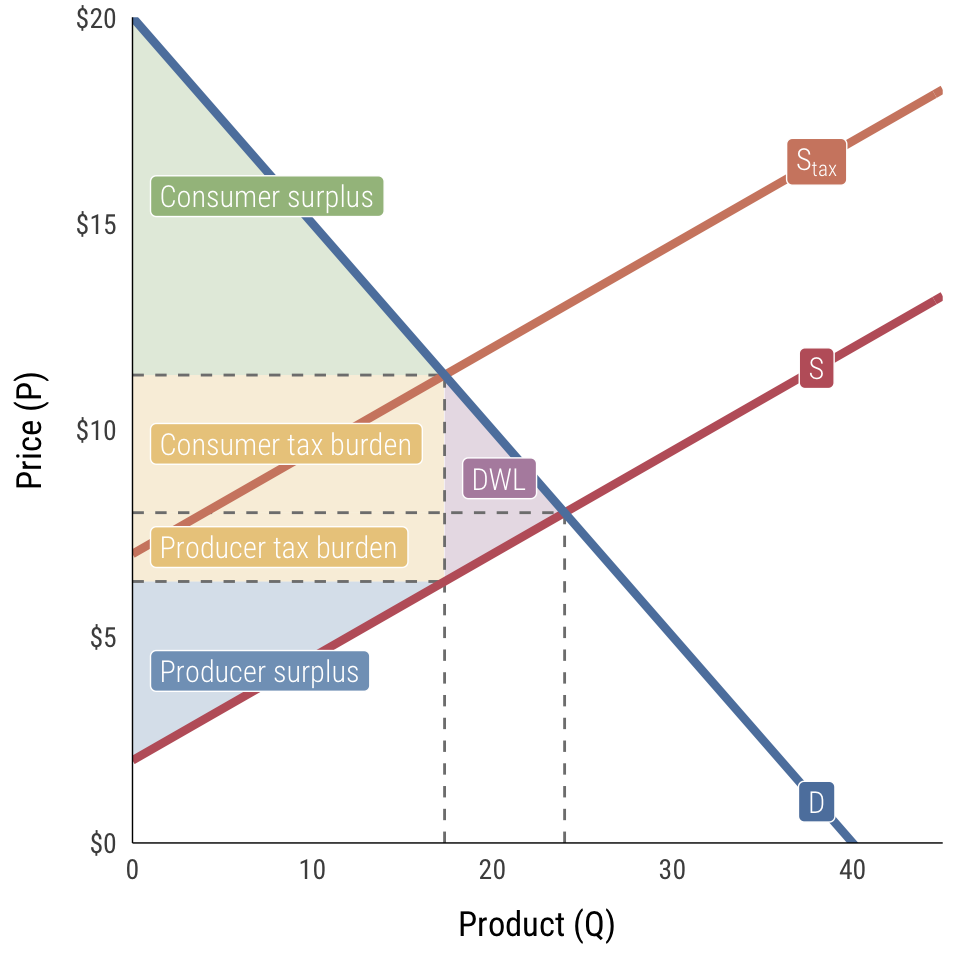# Reference

## Citations and bibliography

You can open the file in BibDesk on macOS, JabRef on Windows, or Zotero or Mendeley online.

## Things you should know for Exam 1

### Capitalism

You should understand…

• …the components of the capitalist economic system: private property, markets, and firms
• …what happens when any of these components gets distorted
• …what makes public goods different from regular goods (see public goods game)
• …what institutions are and how they coordinate action
• …what GDP is, what it measures, what it doesn’t measure, what problems there are with it, what alternatives there are for it, and why it continues to be popular
• …the difference between real and nominal values (and why we care)
• …what a price index is
• …what purchasing power parity (PPP) is (and also what the Big Mac Index is)
• …the downsides of capitalism (inequality + environmental damage)

Games and simulations played in class:

• Pit market trading with paper clips: how supply and demand are derived from producer willingness to accept and consumer willingness to pay

Important fomulas:

$\text{Real} = \frac{\text{Nominal}}{\text{Price Index / 100}}$

• Percent change:

$\frac{\text{Current} - \text{Previous}}{\text{Previous}}$

• Compound annual growth rate (CAGR); periodic method (this assumes interest is compounded once a year; this is the harder method and you don’t really need to use it):

$r = exp(\frac{ln(\frac{\text{Price index}_{\text{new}}}{\text{Price index}_{\text{old}}})}{t}) - 1$

• Compound annual growth rate (CAGR): continuous method (this assumes interest is compounded continuously; this is the easier method and you should generally use this):

$r = \frac{ln(\frac{\text{Price index}_{\text{new}}}{\text{Price index}_{\text{old}}})}{t}$

### Social interactions, economic outcomes, and incentives

You should understand…

• …that perfectly rational individual behavior can create irrational and inferior social outcomes
• …how to use game theory to analyze social interactions. In particular, you should be able to define the following: game, zero-sum, Pareto efficiency, Nash equilibrium, pure strategy, mixed strategy, dominant strategy
• …what social dilemmas, collective action problems, and tragedies of the commons are
• …the difference between a stag hunt game and a prisoners dilemma game, why that difference is important, and why stag hunts are possibly a better metaphor for social dilemmas
• …what factors prevent individuals from cooperating, such as uneven payoffs, lack of assurance, preference falsification, dishonesty, and selfishness
• …how to fix collective action problems with altruism, repetition and iteration, infinitization, punishment, norms, and institutions
• …how incentives can get crowded out and distorted when extrinsic rewards or punishments replace intrinsic motivation (i.e. don’t marketize important social relationships; pay enough or don’t pay at all)

Games and simulations played in class:

• Public goods game with black and red cards: free riding, non-rivalry, and non-excludability
• The Evolution of Trust: prisoners dilemmas and optimal responses and strategies

### Public policy for fairness and efficiency

You should understand…

• …the difference between Pareto efficiency and fairness
• …why Pareto efficiency is not necessarily the best standard for measuring the success of a policy
• …how we can measure fairness with substantive standards, procedural standards, and Rawlsian standards
• …how cultural perceptions of luck and fairness shape public policy
• …how ideas of efficiency and fairness apply to international trade
• …how public policy can be used to change the payoffs in games (e.g. making it more expensive to use water and deplete public goods)
• …what elasticity measures (i.e. what it means for something to be inelastic vs. elastic)
• …why good public policies should be a Nash equilibrium
• …how philosophy and research deisgn allow us to estimate causal relationships
• …how difference-in-difference estimation works

Games and simulations played in class

• Veil of ignorance simulation: example of how different policies emerge when decided behind a veil of ignorance

### Work, wellbeing, and scarcity

You should understand…

• …what opportunity costs are and how they influence decision making
• …how to draw a budget line and what budget lines mean
• …how utility is measured and what indifference curves are
• …the difference between the marginal rate of substitution (slope of the indifference curve) and the marginal rate of transformation (slope of the feasible frontier)
• …what it means when marginal product and marginal utility diminish
• …how to find the utility-maximizing level of consumption given preferences and budget constraints
• …the difference between normal and inferior goods
• …what income effects and substitution effects are and how they’re related to government policies

Games and simulations played in class:

• XYZ Airlines: diminishing marginal product

Important formulas:

• All the ways marginal utility (or marginal rate of substitution) can be written:

$MRS = \frac{dy}{dx} = \frac{\Delta y}{\Delta x} = \frac{\text{Price}_x}{\text{Price}_y} = \frac{MU_x}{MU_y} = \frac{\partial u / \partial x}{\partial u / \partial y}$

### The firm

You should understand…

• …how the decision-making structures of firms and markets are different
• …that perfectly complete contracts are difficult (if not impossible) to create
• …what happens when there are incomplete contracts
• …what a principal-agent problem is
• …moral hazard
• …how firms can use the labor discipline model to induce higher worker effort
• …why involuntary unemployment is necessary

Games and simulations played in class

• Market for lemons: how adverse selection distorts markets and how the presence of information restores competition to markets

Important fomulas:

• Gini coefficients:

# R code
library(ineq)

# List of incomes
incomes <- c(10000, 20000, 50000, 100000, 200000)

# Calculate Gini coefficient
ineq(incomes, type = "Gini")
##  0.4842105Alternatively, you can use this formula where $$x$$ = income, $$y$$ = cumulative proportion of the population, and $$\mu_x$$ = mean of income:

$\frac{2}{\mu_x} \text{Cov}(x, y)$

### Firms and markets

You should understand…

• …how demand curves are derived from consumer willingness to pay
• …the difference between fixed costs and variable costs
• …how to calculate total cost, total revenue, average fixed costs, average variable costs, marginal cost, marginal revenue, and maximum profit
• …that maximum profit occurs where marginal revenue is equal to marginal cost ($$MR = MC$$)
• …that socially optimal quantity occurs when the demand is equal to the marginal cost ($$\text{demand} = MC$$)
• …how to calculate elasticity of demand ($$-\frac{\Delta Q}{\Delta P} \times \frac{P}{Q}$$)
• …what elasticity measures and why it is important in public policy and administration
• …how a single demand curve can have an overall elasticity and different elasticities at each point
• …economies of scale, diseconomies of scale, economies of agglomeration, network effects, and the difference between short-run and long-run costs
• …that market equilibria (i.e. optimal price and quantity) occur at the intersection of supply and demand curves
• …how government-imposed price floors and price ceilings distort market-clearing equilibria
• …and be able to identify the differences between changes in supply/demand and changes in quantity supplied/demanded
• …what consumer and producer surplus represent
• …the relationship between elasticity of supply and/or demand and the size of consumer and producer surplus
• …how taxes impose deadweight loss on society
• …how the burden of taxes depends on the elasticity of supply and/or demand
• …why governments tax and the philosophical and ethical principles behind who should bear the burden of taxes
• …the difference between price-taking and price-making
• …how firms try to gain market power, including monopolies, branding, cost controls, regulation, and switching costs
• …why firms try to gain market power
• …why firms want to price discriminate
• …the consequences of monopolistic production (lower Q and higher P than what would happen under perfect competition; deadweight loss)
• …how governments can regulate monopolies
• …why natural monopolies exist and how governments can induce them to produce at socially optimal levels
• …how firms need to be somewhat anti-competitive and anti-capitalist in order to maximize profits, innovate, and (essentially) be more competitive and capitalist

Games and simulations played in class:

• BYU Creamery chocolate milk: Q, TVC, TFC, TC, MC, AVC, AFC, ATC, and π

Important fomulas:

• Demand:

$P = aQ + b$

• Total cost:

$TC = TFC + TVC \\ \text{or} \\ \text{A formula using } Q \text{, like} \\ TC = aQ^2 + b$

• Average cost:

$AC = \frac{TC}{Q}$

• Marginal cost:

\begin{aligned} MC &= \frac{\Delta TC}{\Delta Q} \\ &\text{or} \\ MC &= \text{First derivative of TC} \\ &= 2aQ \text{ (if } TC = aQ^2 + b) \end{aligned}

• Total revenue:

\begin{aligned} TR &= PQ \\ &\text{or} \\ TR &= (aQ + b)Q \\ &= aQ^2 + bQ \end{aligned}

• Average revenue:

$AR = \frac{TR}{Q}$

• Marginal revenue:

\begin{aligned} MR &= \frac{\Delta TR}{\Delta Q} \\ &\text{or} \\ MR &= \text{First derivative of TR} \\ &= 2aQ + b \text{ (if } TR = aQ^2 + bQ) \end{aligned}

• Maximum profit:

$max(\pi): MC = MR$

• Price elasticity of demand (see class 15 for an explanation of how to get to $$- \frac{\Delta Q}{\Delta P} \times \frac{P}{Q}$$):

$\varepsilon = -\frac{\% \text{ change in quantity demand}}{\% \text{ change in price}} = - \frac{\Delta Q}{\Delta P} \times \frac{P}{Q}$

Important graphs:

• Consumer surplus, producer surplus, tax revenues, tax burdens, and deadweight loss (use algebra and geometry to figure out the areas of the triangles ($$\frac{1}{2} \times b \times h$$) and rectangles ($$l \times w$$)):## Things you should know for Exam 2

### Institutions, power, and inequality

You should understand…

• …why institutions matter for public administration, policy, and governance
• …the strengths and weaknesses of and the and general differences between three main theoretical approaches to institutions: institutions as rational behavior, institutions as constraints, and institutions as temporary equilibria
• …the difference between informal and formal institutions
• …why informal institutions exert influence over our actions even if they’re not officially codified
• …the difference between self-enforcing, self-reinforcing, and self-undermining institutions
• …the role of path dependency in the emergence of institutions
• …how inequality is measured and why we should care about inequality (Gini coefficients vs. 90:10 ratios)
• …how to calculate absolute and comparative advantage and determine the potential gains from trade

Games and simulations played in class

• Pólya’s Urn: example of path dependency
• International trade game: example of natural institutional emergence, the importance of initial allocations of resources, and the distribution of bargaining power

### Market and government failures

You should understand…

• …the difference between private goods, club goods, common pool resources, and public goods (and how they can be classified as (non)rivalrous and (non)excludable)
• …what a market failure is
• …what a government failure is
• …what public goods are and how governments, the private sector, and the third sector can address them
• …what common pool resources (CPRs) are and how governments, the private sector, and the third sector can address them
• …the difference between social and private marginal cost (supply) and marginal benefit (demand)
• …what externalities are and how governments, the private sector, and the third sector can address them
• …what Coasian bargaining is, when it is advantageous, and why it sometimes fails
• …how cap and trade systems can fix externalities (and when they can’t)
• …how Pigouvian taxes can fix externalities (and when they can’t)
• …how regulation can fix externalities (and when and why it can’t)Remember the peanut butter regulation story—regulation is never strictly economic or mathematical

• …why not everything should be a market
• …why large groups suffer from free riding and how they work to behave like small groups
• …how small factions threaten democracy, but also how they enable it
• …how voting can suffer from failures (Condorcet’s paradox)
• …the difference between income and assets
• …why shared national identity and strong horizontal networks of institutions are important for a country’s social and economic wellbeing
• …how inequitable public policies lead to decreased public goods provision, unequal institutional access, and increased ethnofractionalization
• …why slavery has had lasting institutional impacts on the economic system of today and how government policies have contributed to these consequences (specifically in housing and education)